Definitions of Square Dance Calls and Concepts

Index -->  Plus  |  A1  |  A2  |  C1  |  C2  |  C3A  |  C3B  |  C4  |  NOL  |
Definitions (Text Only) -->  Plus  |  A1  |  A2  |  C1  |  C2  |  C3A  |  C3B  |  C4  |  NOL  |
 Find call:

 \$B8@8l(B

A Diagonal Box is a 2 x 2 which is offset by two positions. EN: 10
Diagonal Box \$B\$O(B 2 position \$B\$:\$l\$?(B 2 x 2 \$B\$G\$9(B. JP: 10

Diagonal Box Formation: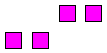\$B\$^\$?\$O(B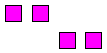From a 2 x 4 there are two Diagonal Boxes. Work in the Distorted 2 x 2 illustrated below. Use Stars for the traffic pattern when appropriate. EN: 20
2 x 4 \$B\$+\$i\$O(B 2 \$B\$D\$N(B Diagonal Box \$B\$,\$"\$j\$^\$9(B. \$B0J2<\$K<(\$9\$h\$&\$K(B Distorted (\$B\$f\$,\$s\$@(B) 2 x 2 \$B\$GF0\$-\$^\$9(B. \$BDL\$jJ}\$H\$7\$F(B Star \$B\$,\$U\$5\$o\$7\$\$\$H\$-\$O(B Star \$B\$r;H\$\$\$^\$9(B.  JP: 20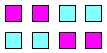Work with these dancers in a Diagonal Box. EN: 30 Diagonal Box \$B\$G\$O(B, \$B\$3\$l\$i\$N?MC#\$GF0\$-\$^\$9(B. JP: 30

Diagonal Box Counter Rotate 1/4: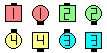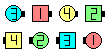Diagonal Box Counter Rotate 1/4\$B\$NA0(B \$B8e(B

\$BCm
• For historical reasons the word 'Box' is often omitted (e.g., 'Diagonal Scoot Back' means 'Diagonal Box Scoot Back'; 'Diagonal Circulate' means 'Diagonal Box Circulate'). EN: 40
\$BNr;KE*\$JM}M3\$G(B 'Box' \$B\$N8@MU\$,\$7\$P\$7\$P>JN,\$5\$l\$^\$9(B (\$BNc\$(\$P(B, 'Diagonal Scoot Back' \$B\$O(B 'Diagonal Box Scoot Back' \$B\$r0UL#\$7(B, 'Diagonal Circulate' \$B\$O(B 'Diagonal Box Circulate' \$B\$r0UL#\$7\$^\$9(B). JP: 40
• Diagonal Coordinate | Motivate | Percolate | Perk Up | etc. means to replace the first Circulate of the call with a Diagonal Box Circulate. It doesn't mean to do the entire call within your Diagonal Box! Nowadays, callers can be more explicit by saying something like Initially Diagonal Box MotivateEN: 50
Diagonal Coordinate | Motivate | Percolate | Perk Up | etc. \$B\$O(B, \$B%3!<%k\$N:G=i\$N(B Circulate \$B\$r(B Diagonal Box Circulate \$B\$GCV\$-49\$(\$k\$3\$H\$r0UL#\$7\$^\$9(B. \$B%3!<%kA4It\$r<+J,\$N(B Diagonal Box \$B\$G9T\$&\$H\$\$\$&\$3\$H\$G\$O\$"\$j\$^\$;\$s(B! \$B:G6a%3!<%i!<\$O(B, Initially Diagonal Box Motivate \$B\$N\$h\$&\$K(B, \$B\$h\$j\$O\$C\$-\$j\$H8@\$&\$3\$H\$,\$"\$j\$^\$9(B. JP: 50
• Diagonal Boxes can show up in formations other than a 2 x 4: EN: 60
Diagonal Boxes 2 x 4 \$B\$G\$J\$\$(B formation \$B\$+\$i\$b;H\$(\$^\$9(B: JP: 60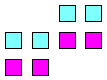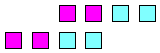(Sometimes called Twin Parallelogram) EN: 70 (\$B\$"\$k\$H\$-\$O(B Twin Parallelogram \$B\$H\$b%3!<%k\$5\$l\$^\$9(B) JP: 70 (Also known as Parallelogram Split) EN: 80 (Parallelogram Split \$B\$HF1\$8\$G\$9(B) JP: 80

\$BDiagonal Column | Line | Wave CONCEPT [C4].Choreography for Diagonal Box ConceptComments? Questions? Suggestions?